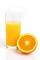# Angle + cylinder - math problems

#### Number of problems found: 7

• A cylinderA cylinder 108 cm high has a circumference of 24 cm. A string makes exactly 6 complete turns around the cylinder while its two ends touch the top and bottom. (forming a spiral around the cylinder). How long is the string in cm?
• Glass of juiceGlass of juice shaped cylinder 16 cm height and base diameter of 7 cm is filled with juice so that the level is 4 cm below the rim of the glass. Determine the maximum angle of the cup can be tilted and juice don't overflow.
• Total displacementCalculate total displacement of the 4-cylinder engine with the diameter of the piston bore B = 6.6 cm and stroke S=2.4 cm of the piston. Help: the crankshaft makes one revolution while the piston moves from the top of the cylinder to the bottom and back t
• Maximum of volumeThe shell of the cone is formed by winding a circular section with a radius of 1. For what central angle of a given circular section will the volume of the resulting cone be maximum?
• Hexagon rotationA regular hexagon of side 6 cm is rotated through 60° along a line passing through its longest diagonal. What is the volume of the figure thus generated?
• Two bodiesThe rectangle with dimensions 8 cm and 4 cm is rotated 360º first around the longer side to form the first body. Then, we similarly rotate the rectangle around the shorter side b to form a second body. Determine the ratio of surfaces of the first and seco
• Circular poolThe base of the pool is a circle with a radius r = 10 m, excluding a circular segment that determines the chord length 10 meters. The pool depth is h = 2m. How many hectoliters of water can fit into the pool?

We apologize, but in this category are not a lot of examples.
Do you have an interesting mathematical word problem that you can't solve it? Submit a math problem, and we can try to solve it.

We will send a solution to your e-mail address. Solved examples are also published here. Please enter the e-mail correctly and check whether you don't have a full mailbox.

Please do not submit problems from current active competitions such as Mathematical Olympiad, correspondence seminars etc...

Angle Problems. Cylinder Problems.